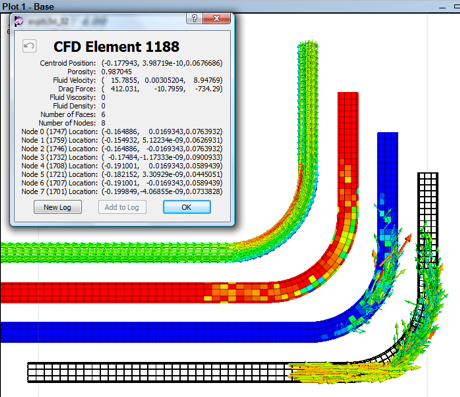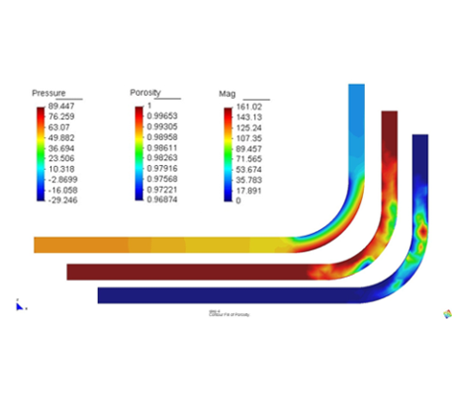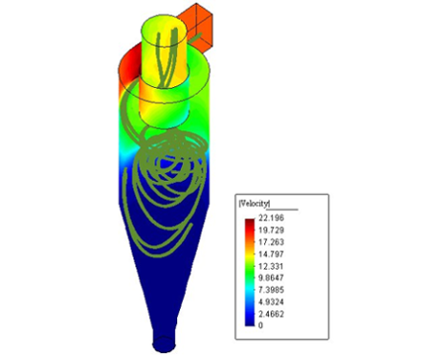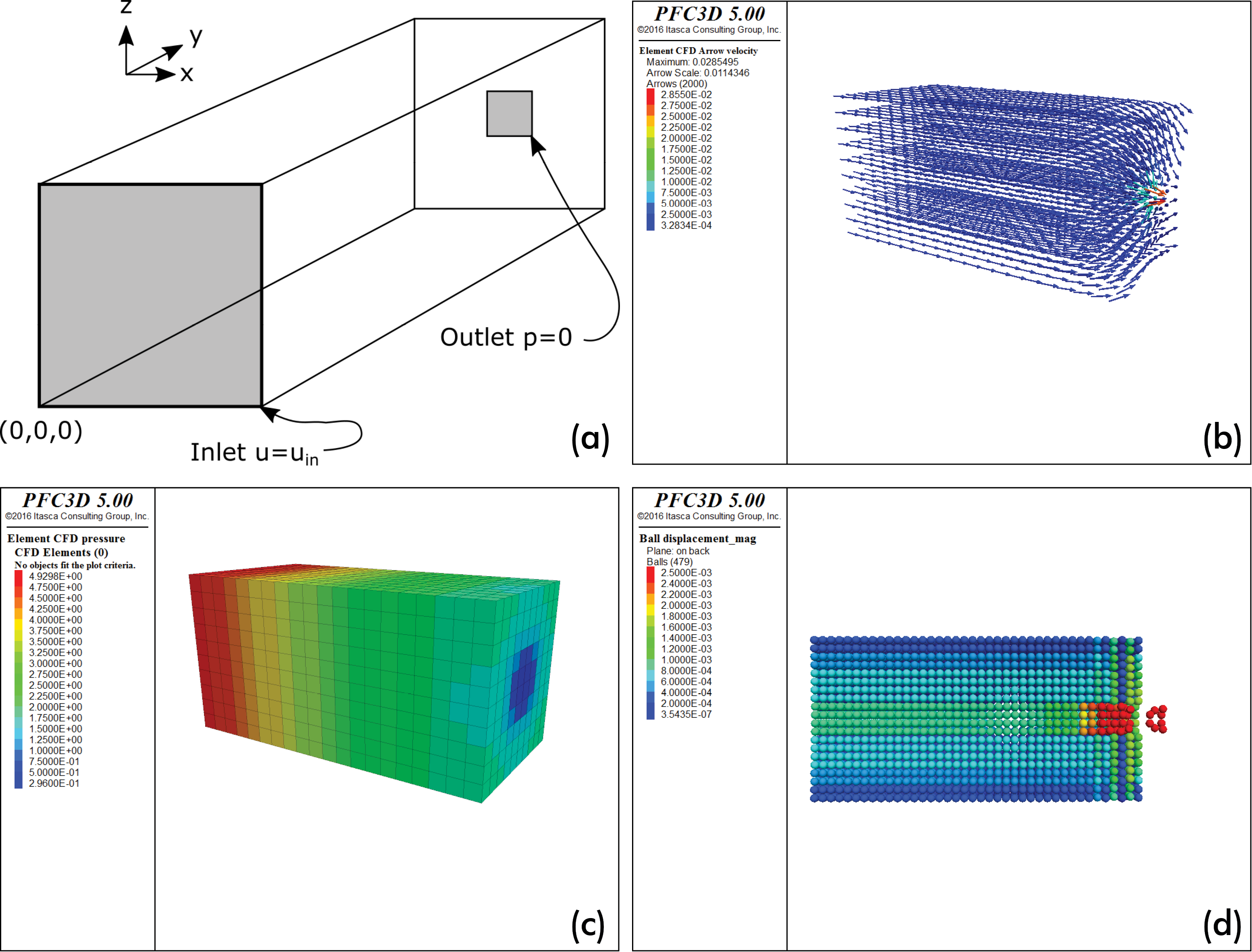## Fluid Dynamics in PFC

### Computational Fluid Dynamics (CFD)

The Computational Fluid Dynamics Module (CFD Module) for PFC3D solves some fluid-particle interaction problems. The module utilizes the volume-averaged coarse-grid approach originally described by (Tsuji et al., 1993); not all fluid-particle interaction problems can be solved with this coarse-grid method.

The CFD Module provides commands and scripting functions to connect to CFD software and solve fluid problems — note a CFD solver is not a built-in component of the module. This gives users the flexibility of coupling PFC3D with the CFD solver of their choice. For an overview of other DEM/fluid coupling methods and applications, see Furtney et al., 2013.PFC3D plot items showing ball velocity vectors and CFD element properties.Color contours of fluid pressure, porosity, and drag force for flow in a bent pipe.

In the coarse-grid approach, equations describing the fluid-flow are solved numerically on a set of elements that are larger than the PFC3D particles. The force acting on the particles due to the fluid is assigned locally to each particle and is based on the fluid conditions in the fluid element that the particle occupies. The formulation for fluid-particle interaction force is accurate and smoothly varying for the practical ranges of porosity and Reynolds numbers (turbulent effects are included in the fluid-particle interaction term).Contours of fluid pressure and particle paths in a cyclone separator.

A corresponding body force is applied to the fluid as an average over one fluid element. Porosity and fluid drag force are calculated from averages of the particle properties in each fluid element. Two-way coupling is realized by periodically exchanging this information between PFC3D and the fluid-flow solver. The synchronization and exchange of information between PFC3D and the fluid-flow software is typically done via TCP socket communication.

The length-scale of fluid-flow structures that can be studied with this method are larger than the PFC particles. Any continuum based fluid-flow model can be used with PFC3D, including the Navier-Stokes equations, potential flow, and the Euler equations. The assumptions that PFC3D makes are: (i) that the fluid elements are larger than the PFC3D particles, (ii) that fluid properties are piecewise linear over the fluid elements and (iii) that the fluid elements do not move.

The CFD Module for PFC3D provides methods to:

• store fluid velocity, fluid pressure, fluid pressure gradient, fluid viscosity and fluid density in each fluid element,
• calculate porosity, and
• automatically apply fluid-particle interaction forces to particles during PFC3D cycling.

The module comes with three examples.

1. One-way coupling of particles falling under gravity in a still fluid.
2. Two-way coupling of low Reynolds number porous flow in a granular material.
3. Two-way coupling with an open-source Navier-Stokes solver.PFC3D example for a porous flow showing the (a) problem schematic for where fluid enters the domain at y=0 and flows out a small outlet at y=1, (b) fluid velocity vectors, (c) contours of fluid pore pressure, and (d) contours of particle displacement.

### References

Tsuji, Y., T. Kawaguchi & Tanata, T. (Discrete Particle Simulation of Two-Dimensional Fluidized Bed), Powder Tech., 77, 79-87 (1993).

Furtney, J. K., F. Zhang and Y. Han. Review of Methods and Applications for Incorporating Fluid Flow in the Discrete Element Method, Proceedings, The Fifth International FLAC/DEM Symposium, (Hangzhou, P.R. China, October, 2013). Minneapolis, Itasca International Inc. (2013).